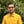Trusted answers to developer questions
Trusted Answers to Developer Questions

Related Tags

php
infinite
math
communitycreator

# What is is_infinite() in PHP?Harris Amjad

Grokking Modern System Design Interview for Engineers & Managers

Ace your System Design Interview and take your career to the next level. Learn to handle the design of applications like Netflix, Quora, Facebook, Uber, and many more in a 45-min interview. Learn the RESHADED framework for architecting web-scale applications by determining requirements, constraints, and assumptions before diving into a step-by-step design process.

The is_infinite() function in PHP returns True (1) if the value is infinite. Otherwise, it returns False (nothing). In other words, this function is used to check if the number is infinite or not.

Figure 1 shows the visual representation of the is_infinite() function.

Figure 1: Visual representation of the is_infinite() function

## Syntax

bool is_infinite(number)


## Parameter

This function requires the number as a parameter.

## Return value

This function returns True (1) if the value is infinite. Otherwise, it returns False (nothing).

## Example

The following example shows how to use is_infinite() in PHP.

<?php#number echo("is_infinite(10): ");echo (is_infinite(10));echo("\n");echo("is_infinite(5.3): ");echo (is_infinite(5.3));echo("\n");echo("is_infinite(-5.3): ");echo (is_infinite(-5.3));echo("\n");echo("is_infinite(0): ");echo (is_infinite(0));?>	<?php#infinityecho("is_infinite(log(0)): ");echo (is_infinite(log(0)));echo("\n");echo("is_infinite(INF): ");echo (is_infinite(INF));echo("\n");echo("is_infinite(-INF): ");echo (is_infinite(-INF));?>

RELATED TAGS

php
infinite
math
communitycreator

CONTRIBUTORHarris Amjad

Grokking Modern System Design Interview for Engineers & Managers

Ace your System Design Interview and take your career to the next level. Learn to handle the design of applications like Netflix, Quora, Facebook, Uber, and many more in a 45-min interview. Learn the RESHADED framework for architecting web-scale applications by determining requirements, constraints, and assumptions before diving into a step-by-step design process.

Keep Exploring

Learn in-demand tech skills in half the time